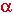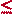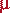Math & Science Home | Proficiency Tests | Mathematical Thinking in Physics | Aeronauts 2000

 CONTENTS Introduction Fermi's Piano Tuner Problem How Old is Old? If the Terrestrial Poles were to Melt... Sunlight Exerts Pressure Falling Eastward What if an Asteroid Hit the Earth Using a Jeep to Estimate the Energy in Gasoline How do Police Radars really work? How "Fast" is the Speed of Light? How Long is a Light Year? How Big is a Trillion? "Seeing" the Earth, Moon, and Sun to Scale Of Stars and Drops of Water If I Were to Build a Model of the Cosmos... A Number Trick Designing a High Altitude Balloon Pressure in the Vicinity of a Lunar Astronaut Space Suit due to Outgassing of Coolant Water Calendar Calculations Telling Time by the Stars - Sidereal Time Fields, an Heuristic Approach The Irrationality ofThe Irrationality ofThe Number (i)i Estimating the Temperature of a Flat Plate in Low Earth Orbit Proving that (p)1/n is Irrational when p is a Prime and n>1 The Transcendentality ofIdeal Gases under Constant Volume, Constant Pressure, Constant Temperature and Adiabatic Conditions Maxwell's Equations: The Vector and Scalar Potentials A Possible Scalar Term Describing Energy Density in the Gravitational Field A Proposed Relativistic, Thermodynamic Four-Vector Motivational Argument for the Expression-eix=cosx+isinx Another Motivational Argument for the Expression-eix=cosx+isinx Calculating the Energy from Sunlight over a 12 hour period Calculating the Energy from Sunlight over actual full day Perfect Numbers-A Case Study Gravitation Inside a Uniform Hollow Sphere Further note on Gravitation Inside a Uniform Hollow Sphere Pythagorean Triples Black Holes and Point Set Topology Additional Notes on Black Holes and Point Set Topology Field Equations and Equations of Motion (General Relativity) The observer in modern physics A Note on the Centrifugal and Coriolis Accelerations as Pseudo Accelerations - PDF File On Expansion of the Universe - PDF File

## A Number Trick

Select a three digit number in which the first and the last digit differ by at least two. Construct a second number by reversing the order of digits in the first. Form a third number by taking the difference of the first two. Reverse the order of digits in the third number to construct a fourth, and add the third and fourth number. The result is the number 1089.

Example: Select the number 732. Note: 7 - 2 = 5 > 2, making this number a valid choice. Next, construct the number 237 by reversing the order of digits. Take the difference:

732 - 237 = 495

From 495, construct the number 594, and add:

495 + 594 = 1089.

Proof: Let the originally selected number be represented by

 100a + 10b + c 1

where a, b, c, are integers between 0 and 9 inclusive, and occupy the hundreds, tens, and units places, respectively. We may assume, without loss of generality, that a > c. Now, construct a new number by reversing the order of digits

 100c + 10b + a 2

Take the difference

 100(a - c) + (c - a) 3

and simplify to

 99(a - c) = 994

where= a - c. We would like to represent this number, algebraically, as a number in base ten; i.e., with a form similar to that shown in 1. We begin by rewriting 99as

 99= (10 . 9) + (1 . 9) 5

Since 29 by hypothesis, we know that 18981 so that we may write

 9= 10+ v 6

whereand v are integers between 0 and 9 inclusive, and occupy the tens and units places, respectively. Thus, the Right Hand Side of eq. 5 may be rewritten as

(10 . 9) + (1 . 9) = [10 . (10+ v)] + [1 . (10+ v)]
 = 100+ 10(+ v) + v 7

If the integer+ v does not exceed a single digit, then the number,

100+ 10(+ v) + v

is the number sought. Let us find all possible values of+ v for 29:9+ v 2 18 9 3 27 9 4 36 9 5 45 9 6 54 9 7 63 9 8 72 9 9 81 9

Thus,+ v does not exceed 9 (in fact, it equals 9 in all cases). We conclude that the number, 100+ 10(+ v) + v, is the one we sought, and correctly represents the difference, 99, as a number in base ten, withoccupying the hundreds place; (+ v), the tens place; and v, the units place. The number obtained by reversing the order of its digits is then

 100v + 10(+ v) +8

and the sum of these last two numbers is

 100(+ v) + 20(+ v) + (+ v) = 121(+ v) 9

But, for all values of a considered, (+ v)= 9, so that 121(+ v) = 1089.

Please send suggestions/corrections to:
Web Related: David.Mazza@grc.nasa.gov
Technology Related: Joseph.C.Kolecki@grc.nasa.gov
Responsible NASA Official: Theresa.M.Scott (Acting)